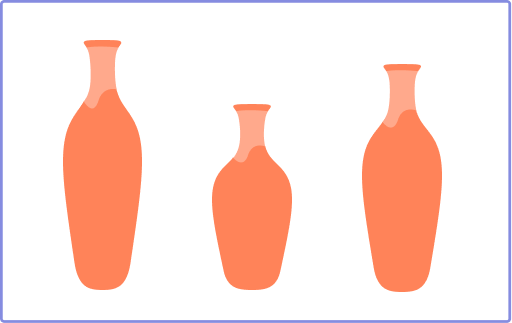# Shortest in Math – Definition, Examples, Facts

Home » Math-Vocabluary » Shortest in Math – Definition, Examples, Facts

## What Is ‘Shortest’ in Math?

“Shortest” simply refers to the smallest in length or height. It helps us understand and compare measurements like length, height, or distance.

We use different words to compare quantities, such as:

short and long to compare lengths.

small and big to compare size.

short and tall to compare heights.

The term ‘shortest’ refers to the least length, height, or distance. It can also refer to the least period of time or a way of doing something that takes the least time.

## Shortest: Definition

“Shortest” is the word used to describe an object with the smallest length, height, or distance.

For example, we use the word ‘shortest’ in the sentence like ‘the shortest pencil’ or ‘the shortest kid in the class’ or ‘the shortest road’. We sometimes use it to describe the least amount of time. For example, we can say ‘the shortest period or duration’.

## Real Life Example to Identify the Shortest Object

Take a look at the image above. What is the shortest object here?

Clearly, it is the pen since it is the smallest in length.

## Shortest in Length

Let’s take a look at the given image. There are three pencils. Comparing the lengths, we can say that the GREEN pencil is the shortest in length.

On the other hand, the PINK one is the longest of the three and the BLUE pencil is shorter than the PINK one.

## Shortest in Height

There are four blocks shown in the given image. Comparing their heights, we can see that the RED one is the shortest of them all in height.

## Shortest in Distance

Consider an example in geometry. The distance of the point P and the line l can be drawn using many different line segments, but the shortest distance among them is the perpendicular PD. So, the shortest distance of a point to a line is the perpendicular distance.

## How to Use Shortest in a Sentence?

Here are some “shortest examples:

• The shortest way to the supermarket is the main street.
• The shortest path home is by the central mall.
• The shortest person in the room is standing there.

## Conclusion

Shortest is a concept that helps us understand one quantity with respect to others. It helps us compare the measurements of different objects and quantities and express which is the least!

## Solved Examples On Shortest

1. Heena is 6 feet tall. Alex is 6.3 feet tall. Helen is 5.9 feet tall. Who is the shortest?

Solution:

Helen is the shortest because her height is the least of the three persons.

2. Identify the crayon smallest in length.

Solution:

The blue crayon is the shortest since it is the smallest in length.

3. Jack took 20 minutes to complete a test. Raj took 30 minutes. Linda took 1 hour to complete it. Who finished the test in the shortest time?

Solution:

20 minutes was the least amount of time taken to complete the test.

4. Identify the stop sign that has the least height.

Solution: The first STOP sign is the shortest in height.

## Practice Problems On Shortest

1

### Tim is 5.7 feet tall. Luke is 6 feet tall. Nate is 5.2 feet tall. Who is the shortest?

Tim
Tim and Nate
Nate
Luke
CorrectIncorrect
Comparing the heights, we can say that Nate is the shortest.
2

### Which of the following candles is the tallest?Pink
Blue
Green
None
CorrectIncorrect
The pink candle is the tallest because it has the greatest length.
3

### There are three routes to reach school. One is 3 miles long, the second is 2 miles long, and the third is 1 mile long. If you take the shortest route, how long will the distance be?

2.5 miles
1.5 miles
1 mile
2 miles
CorrectIncorrect
The path that is 1 mile long has the least distance out of the three paths to school.
4

### The middle vase is the _____ among three.shortest
tallest
longest
biggest
CorrectIncorrect
Since the middle vase has the least height, we can say it is the shortest.
5

Zebra
Camel
Rabbit
Horse
CorrectIncorrect